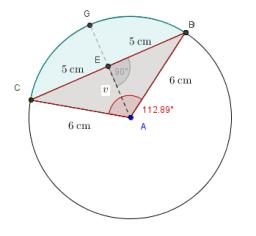# Calculate 4228

A circle k (S, 5cm) is given. Calculate the length of the chord of the circle k if it is 3 cm from the center S.

t =  8 cm

### Step-by-step explanation:Did you find an error or inaccuracy? Feel free to write us. Thank you!

Tips for related online calculators
The Pythagorean theorem is the base for the right triangle calculator.

#### Grade of the word problem:

We encourage you to watch this tutorial video on this math problem: